cancel
Showing results for
Did you mean:Helper III

## need to display latest order date with repective order ID

Hi,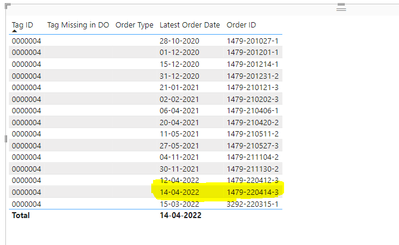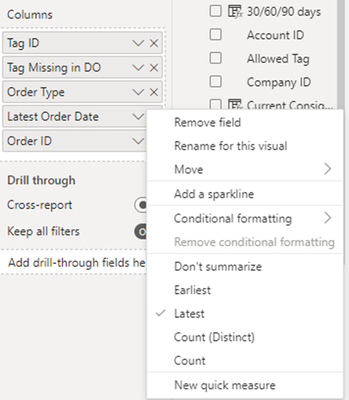I just want to display the latest date (14-04-2022) along with respective Order ID , but while selecting latest in Order ID Column its returning last column value of the that TagId. But actually it should display the Order ID for that date.

I enclosed the images for the reference purpose.

1 ACCEPTED SOLUTIONCommunity Support

Hi @rveerasamy ,

It's because row context in your visual affects the result. I made an example and provide a solution for your reference.

Sample data: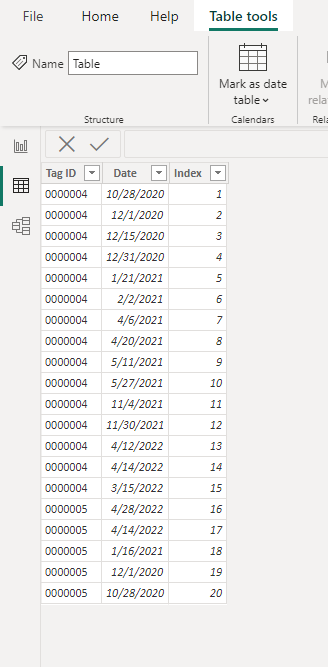If there is only a Group ID in the visual, the latest date will be returned grouped according to the Group ID.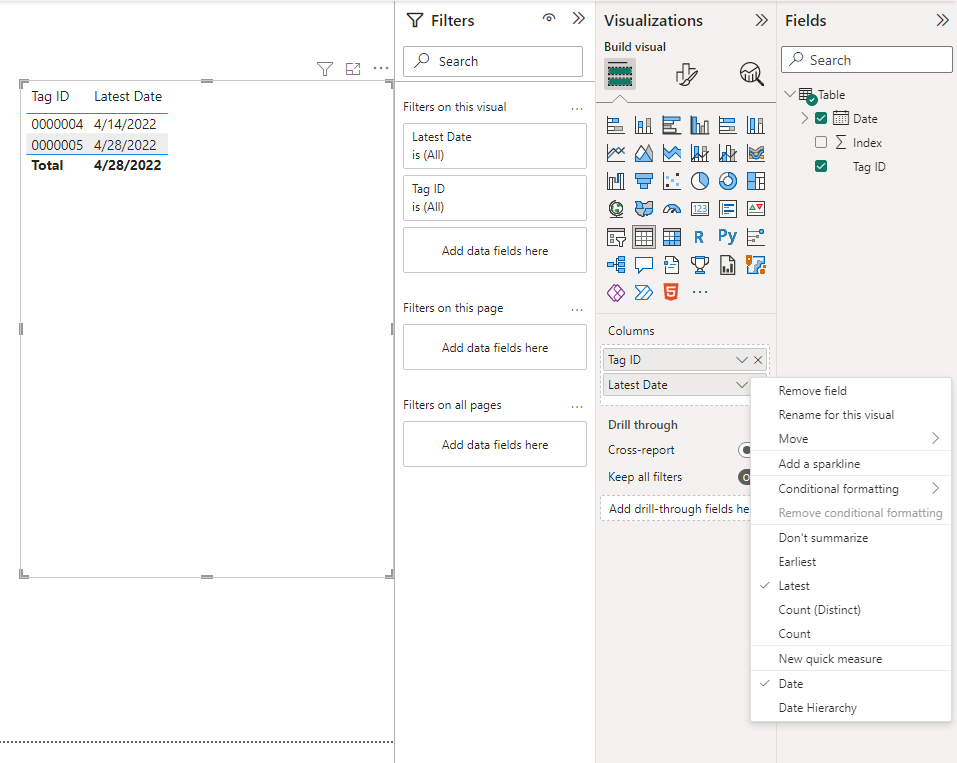If you add an index column at this time, then because of the row context, the result will appear as follows.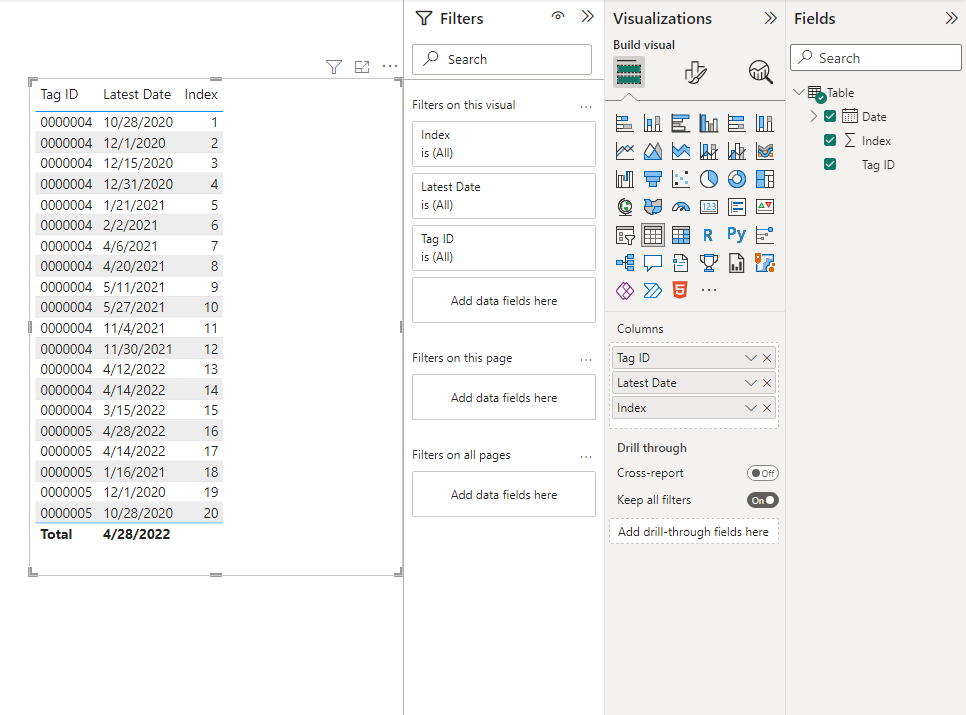Here's the solution. You can create a measure as the visual-level filters.

``````Measure =
VAR _date =
CALCULATE (
MAX ( 'Table'[Date] ),
FILTER ( ALLSELECTED ( 'Table' ), [Tag ID] = MAX ( 'Table'[Tag ID] ) )
)
RETURN
IF ( _date = MAX ( 'Table'[Date] ), 1 )
``````

Put the measure into the visual-level filters and set up show items when the value is 1.

And set the Date field to Don't summarize.Best Regards,

Stephen Tao

If this post helps, then please consider Accept it as the solution to help the other members find it more quickly.

2 REPLIES 2Community Support

Hi @rveerasamy ,

It's because row context in your visual affects the result. I made an example and provide a solution for your reference.

Sample data:If there is only a Group ID in the visual, the latest date will be returned grouped according to the Group ID.If you add an index column at this time, then because of the row context, the result will appear as follows.Here's the solution. You can create a measure as the visual-level filters.

``````Measure =
VAR _date =
CALCULATE (
MAX ( 'Table'[Date] ),
FILTER ( ALLSELECTED ( 'Table' ), [Tag ID] = MAX ( 'Table'[Tag ID] ) )
)
RETURN
IF ( _date = MAX ( 'Table'[Date] ), 1 )
``````

Put the measure into the visual-level filters and set up show items when the value is 1.

And set the Date field to Don't summarize.Best Regards,

Stephen Tao

If this post helps, then please consider Accept it as the solution to help the other members find it more quickly.Solution Sage

To display the latest date along with the respective Order ID, you can use the MAXX function in Power BI. Here's an example DAX formula you can use:

sqlCopy code
Latest Order Date = MAXX(GROUPBY(Orders, Orders[Order ID], "LatestOrderDate", MAX(Orders[Order Date])), [LatestOrderDate])
` `

This formula groups the orders by Order ID and finds the maximum order date for each group. Then, it returns the maximum order date across all groups. You can add this formula to a new card or table in your report to display the latest order date with the respective order ID.